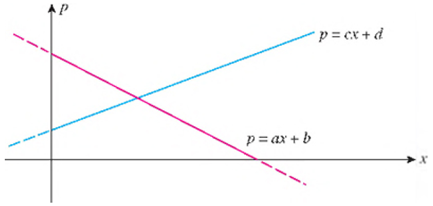Chapter 2.3, Problem 69E

Chapter
Section
Textbook Problem

Supply and Demand Equations Suppose the demand and supply equations for a certain commodity are given by p = ax + b and p = cx + d, respectively, where a < 0, c > 0, and b > d > 0 (see the figure below). a. Find the equilibrium quantity' and equilibrium price in terms of a, b, c, and d. b. Use part (a) to determine what happens to the market equilibrium if c is increased while a, b, and d remain fixed. Interpret your answer in economic terms. c. Use part (a) to determine what happens to the market equilibrium if b is decreased while a, c, and d remain fixed. Interpret your answer in economic terms.(a)

To determine
The equilibrium quantity and equilibrium price.

Explanation

Given information:

The supply equation for a certain commodity is,

p=ax+b

The demand equation for a certain commodity is,

p=cx+d .

Where, a<0,c>0andb>d>0

Definition Used:

When the quantity produced is equal to the quantity demanded, the quantity is known as equilibrium quantity and the corresponding price is known as equilibrium price.

Calculation:

Equate the supply equation and demand equation to evaluate the equilibrium quantity.

Divide both sides by (ca) to evaluate the value of x

(b)

To determine
The effect on market if c is increased and other remains fixed.

(c)

To determine
The effect on market if b is decreased and other remains fixed.

Still sussing out bartleby?

Check out a sample textbook solution.

See a sample solution

The Solution to Your Study Problems

Bartleby provides explanations to thousands of textbook problems written by our experts, many with advanced degrees!

Get Started

916 Sketch the polar curve. r=cos3

Calculus (MindTap Course List)

Evaluate the integral, if it exists. sintcostdt

Single Variable Calculus: Early Transcendentals, Volume I

How do you solve an equation (i) algebraically? (ii) graphically?

Precalculus: Mathematics for Calculus (Standalone Book)

Simplify the expressions in Exercises 97106. 41/147/2

Finite Mathematics and Applied Calculus (MindTap Course List)

Calculate y'. 11. y=xcosx

Single Variable Calculus: Early Transcendentals

Simplify: 1240

Elementary Technical Mathematics